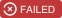Newfoundland Pitbull Mix

# npm

## shamir

0.7.1 • Public • Published

# Shamir's Secret SharingA implementation of Shamir's Secret Sharing algorithm over GF(256) in both Java and JavaScript.

Note: module name for Java 9+ is `com.codahale.shamir`.

## How it works

Shamir's Secret Sharing algorithm is a way to split an arbitrary secret `S` into `N` parts, of which at least `K` are required to reconstruct `S`. For example, a root password can be split among five people, and if three or more of them combine their parts, they can recover the root password.

### Splitting secrets

Splitting a secret works by encoding the secret as the constant in a random polynomial of `K` degree. For example, if we're splitting the secret number `42` among five people with a threshold of three (`N=5,K=3`), we might end up with the polynomial:

``````f(x) = 71x^3 - 87x^2 + 18x + 42
``````

To generate parts, we evaluate this polynomial for values of `x` greater than zero:

``````f(1) =   44
f(2) =  298
f(3) = 1230
f(4) = 3266
f(5) = 6822
``````

These `(x,y)` pairs are then handed out to the five people.

### Joining parts

When three or more of them decide to recover the original secret, they pool their parts together:

``````f(1) =   44
f(3) = 1230
f(4) = 3266
``````

Using these points, they construct a Lagrange polynomial, `g`, and calculate `g(0)`. If the number of parts is equal to or greater than the degree of the original polynomial (i.e. `K`), then `f` and `g` will be exactly the same, and `f(0) = g(0) = 42`, the encoded secret. If the number of parts is less than the threshold `K`, the polynomial will be different and `g(0)` will not be `42`.

### Implementation details

Shamir's Secret Sharing algorithm only works for finite fields, and this library performs all operations in GF(256). Each byte of a secret is encoded as a separate `GF(256)` polynomial, and the resulting parts are the aggregated values of those polynomials.

Using `GF(256)` allows for secrets of arbitrary length and does not require additional parameters, unlike `GF(Q)`, which requires a safe modulus. It's also much faster than `GF(Q)`: splitting and combining a 1KiB secret into 8 parts with a threshold of 3 takes single-digit milliseconds, whereas performing the same operation over `GF(Q)` takes several seconds, even using per-byte polynomials. Treating the secret as a single `y` coordinate over `GF(Q)` is even slower, and requires a modulus larger than the secret.

## Java Performance

It's fast. Plenty fast.

For a 1KiB secret split with a `n=4,k=3` scheme:

``````Benchmark         (n)  (secretSize)  Mode  Cnt     Score    Error  Units
Benchmarks.join     4          1024  avgt  200   196.787 ±  0.974  us/op
Benchmarks.split    4          1024  avgt  200   396.708 ±  1.520  us/op
``````

N.B.: `split` is quadratic with respect to the number of shares being combined.

## JavaScript Performance

For a 1KiB secret split with a `n=4,k=3` scheme running on NodeJS v10.16.0:

``````Benchmark         (n)  (secretSize)  Cnt   Score   Units
Benchmarks.join     4          1024  200   2.08    ms/op
Benchmarks.split    4          1024  200   2.78    ms/op
``````

Split is dominated by the calls to get random polynomials per byte of the secet. Using a more realistic 128 bit secret with `n=4,k=3` scheme running on NodeJS v10.16.0:

``````Benchmark         (n)  (secretSize)  Cnt   Score   Units
Benchmarks.join     5          1024  200   0.083    ms/op
Benchmarks.split    5          1024  200   0.081    ms/op
``````

## Tiered sharing

Some usages of secret sharing involve levels of access: e.g. recovering a secret requires two admin shares and three user shares. As @ba1ciu discovered, these can be implemented by building a tree of shares:

By discarding the third admin share and the first two sets of user shares, we have a set of shares which can be used to recover the original secret as long as either two admins or one admin and three users agree.

## Keywords

### Install

`npm i shamir`

### Repository

github.com/simbo1905/shamir

42

0.7.1